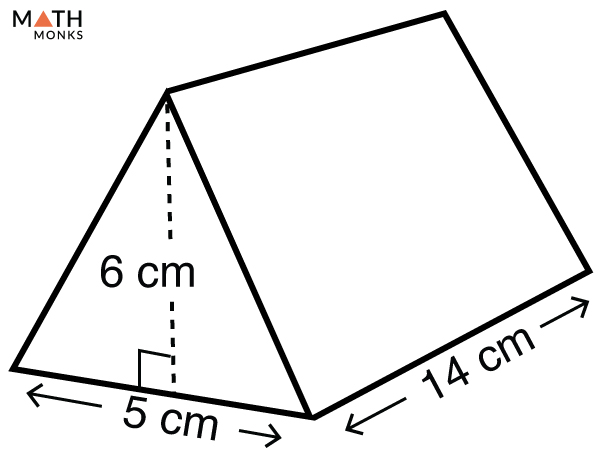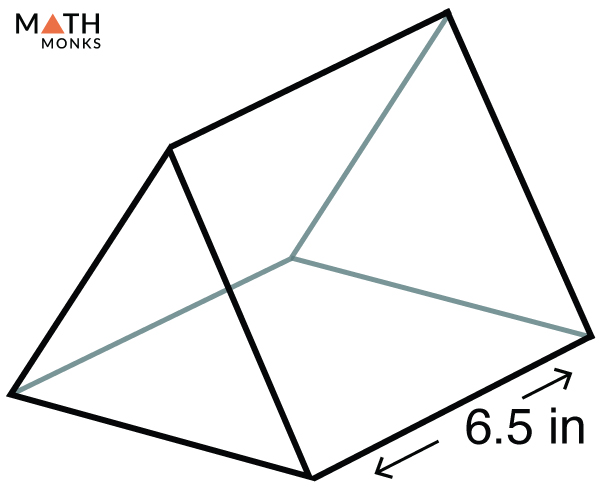# Volume of a Triangular Prism

The volume of a triangular prism is the space it occupies in the three-dimensional plane. It is measured in cubic units such as m3, cm3, mm3, ft3.

## Formula

The formula to calculate the volume of a triangular prism is given below:

In the above formula, the product of the half the base edge and the height is the base area of the prism. Therefore, we can also write the formula as:

Volume (V) = Base Area x Length

Let us solve some examples to understand the above concept better.

## Solved Examples

Find the volume of a right triangular prism whose base is 7 cm, the height is 8 cm, and the length is 25 cm.

Solution:

As we know,
Volume (V) = ${\dfrac{1}{2}\times b\times h\times l}$, here b = 7 cm, h = 8 cm, l = 25 cm
V = ${\dfrac{1}{2}\times 7\times 8\times 25}$
= 700 cm3Find the volume of a triangular prism given in the figure.

Solution:

As we know,
Volume (V) = ${\dfrac{1}{2}\times b\times h\times l}$, here b = 5 cm, h = 6 cm, l = 14 cm
V = ${\dfrac{1}{2}\times 5\times 6\times 14}$
= 210 cm3

Finding the volume of a triangular prism when the BASE AREA is knownFind the volume of a triangular prism given in the figure whose base area is 256 in2.

Solution:

As we know,
Volume (V) = Base Area × Length, here base area = 256 in2, length = 6.5 in
V = 256 × 6.5
= 1664 in3

• More Resources:

1.Kaden Ekstrum says: